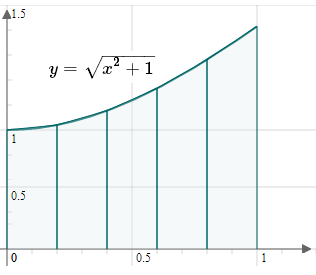Search IntMath
Close

450+ Math Lessons written by Math Professors and Teachers

5 Million+ Students Helped Each Year

1200+ Articles Written by Math Educators and Enthusiasts

Simplifying and Teaching Math for Over 23 Years

# 5. The Trapezoidal Rule

by M. Bourne

### Interactive exploration

See an applet where you can explore Simpson's Rule and other numerical techniques:

Problem: Find

int_0^1sqrt(x^2+1)\ dx

We put u = x^2+ 1 then du = 2x\ dx.

But the question does not contain an x\ dx term so we cannot solve it using any of the integration methods we have met so far.

We need to use numerical approaches. (This is usually how software like Mathcad or graphics calculators perform definite integrals).

We can use one of two methods:

## The Trapezoidal Rule

We saw the basic idea in our first attempt at solving the area under the arches problem earlier.

Instead of using rectangles as we did in the arches problem, we'll use trapezoids (trapeziums) and we'll find that it gives a better approximation to the area.

y0
Deltax
y1
Deltax
y2
Deltax
y3
Deltax
y4
Deltax
y5
Deltax
y6

Area under curve using trapezoidal Rule

The approximate area under the curve is found by adding the area of all the trapezoids.

(Recall that we write "Δx" to mean "a small change in x".)

### Area of a trapezoid

p
q
h
"Area"=h/2(p+q)

Area of a trapezoid (trapezium)

Now, the area of a trapezoid (trapezium) is given by:

"Area"=h/2(p+q)

We need "right" trapezoids (which means the parallel sides are at right angles to the base), and they are rotated 90° so that their new base is actually h, as follows, and h = Δx.

y0
y1
Deltax

A "typical" trapezoid

So the total area is given by:

"Area"~~1/2(y_0+y_1)Deltax+ 1/2(y_1+y_2)Deltax+ 1/2(y_2+y_3)Deltax+...

We can simplify this to give us the Trapezoidal Rule, for n trapezoids:

"Area"~~ Deltax((y_0)/2+y_1+y_2+y_3+ {:...+(y_n)/2)

To find Δx for the area from x=a to x=b, we use:

Deltax=(b-a)/n

and we also need

y_0= f(a)

y_1= f(a + Δx)

y_2= f(a + 2Δx)

...

y_n= f(b)

### Note

• We get a better approximation if we take more trapezoids [up to a limit!].
• The more trapezoids we take, Delta x will tend to 0, that is, Δx → 0.
• We can write (if the curve is above the x-axis only between x=a and x=b):

"Area"=int_a^b f(x)dx

~~ Deltax((y_0)/2+y_1+{:...+(y_n)/2)

## Don't miss...

There is an interactive applet where you can explore the Trapezoid Rule, here:

Calculus from First Principles applet

## Exercise

Using n= 5, approximate the integral:

int_0^1sqrt(x^2+1)\ dx

This is the situation:I have joined each of the points at the top of the vertical segments with a straight line.

Here, a = 0 and b = 1, and the width of each trapezoid is given by:

Delta x=frac{b-a}{n}=frac{1-0}{5}=0.2

y_0= f(a)= f(0)=sqrt{0^2+1}=1

y_1= f(a + Δx)= f(0.2)=sqrt{0.2^2+1}=1.0198039

y_2= f(a + 2Δx)= f(0.4)=sqrt{0.4^2+1}=1.0770330

y_3= f(a + 3 Δx)= f(0.6)=sqrt{0.6^2+1}=1.1661904

y_4= f(a + 4 Δx)= f(0.8)=sqrt{0.8^2+1}=1.2806248

y_5= f(a + 5 Δx)= f(1.0)=sqrt{1^2+1}=1.4142136

So we have:

"Integral" ≈

0.2(1/2 times 1+1.0198039 +1.0770330+1.1661904 {:+1.2806248+1/2times 1.4142136)

=1.150

So int_0^1 sqrt{x^2+1}\ dx ≈ 1.150

We can see in the graph above the trapezoids are very close to the original curve, so our approximation should be close to the real value. In fact, to 3 decimal places, the integral value is 1.148.

## Problem SolverThis tool combines the power of mathematical computation engine that excels at solving mathematical formulas with the power of GPT large language models to parse and generate natural language. This creates math problem solver thats more accurate than ChatGPT, more flexible than a calculator, and faster answers than a human tutor. Learn More.